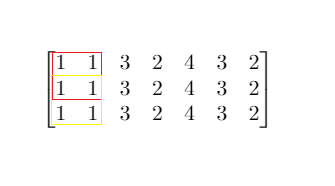## Saturday, January 25, 2020

### Problem Statement

Given a `m x n` matrix `mat` and an integer `threshold`. Return the maximum side-length of a square with a sum less than or equal to `threshold` or return 0 if there is no such square.

Example 1:```Input: mat = [[1,1,3,2,4,3,2],[1,1,3,2,4,3,2],[1,1,3,2,4,3,2]], threshold = 4
Output: 2
Explanation: The maximum side length of square with sum less than 4 is 2 as shown.
```
Example 2:
```Input: mat = [[2,2,2,2,2],[2,2,2,2,2],[2,2,2,2,2],[2,2,2,2,2],[2,2,2,2,2]], threshold = 1
Output: 0
```
Example 3:
```Input: mat = [[1,1,1,1],[1,0,0,0],[1,0,0,0],[1,0,0,0]], threshold = 6
Output: 3
```
Example 4:
```Input: mat = [[18,70],[61,1],[25,85],[14,40],[11,96],[97,96],[63,45]], threshold = 40184
Output: 2
```

Constraints:
• `1 <= m, n <= 300`
• `m == mat.length`
• `n == mat[i].length`
• `0 <= mat[i][j] <= 10000`
• `0 <= threshold <= 10^5`

### Video Tutorial

You can find the detailed video tutorial here

### Thought Process

There is an absolute brute force way is to calculate the sum of each square in every iteration. There are previous similar problems that we can have a prefix sum matrix, similar to the rolling sum idea on one dimensional array.

A prefix sum matrix is a matrix that for each cell[i, j] represents the sum in original matrix from matrix[0, 0] to matrix [i, j] (inclusive)

The advantage is later we can get any rectangle sum simply performing below

sum from [i, j] to [i + len][j + len] = prefixSum[i + len][j + len] - prefixSum[i - 1][j + len] - prefixSum[i + len][j - 1] + prefixSum[i - 1][j - 1]
Two more optimizations we can do
• We can apply binary search instead of a linear one to find the max length
• We can also keep a record of the sum while building up the prefix sum matrix. The reason this works is if it has a square say 3x3 that meets the requirement, then it would definitely have a square 2x2 meet the requirement. So we can start from 1x1, 2x2 till we reach the max. Pretty smart! Kudos to @drunkpiano

### Solutions

#### Prefix sum implementation

Time Complexity: O(M*N*min(M,N)) where M is row N is col, essentially O(N^3)
Space Complexity: O(M*N) since we need

References

#### 1 comment:

1.Thanks. It was very helpful. Your diagrams really helped in understanding. I've a little doubt. In that Brute Force Solution, why are returning len+1 instead of len?

> Thank you for your reply and that's a good catch. You can think of Len as the delta distance, as a matter of fact, I would probably rename length as delta, and star the for loop with
for (int len = Math.min(row, col) ; len >= 1; len--) -> for (int len = Math.min(row, col) - 1; len >= 1; len--)

Thank your for your comment! Check out us at https://baozitraining.org/ if you need mock interviews!